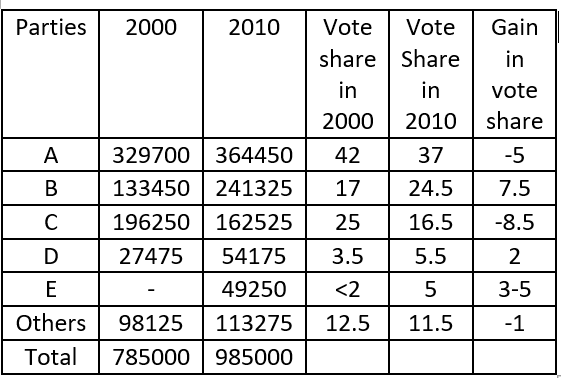### XAT 2015 Question 83

Instructions

Answer the questions on the basis of information given below.

Twitter allows its users to post/share and read short messages known as tweets. Tweets can be of three types – Positive Tweets (in support), Negative Tweets (against) and Neutral Tweets. The following table presents the Number of Votes and Tweets received by certain political parties.

* Any party which has secured less than 2% of the total votes falls under ‘Other Parties’ category. For example, Party E secured less than 2% of total votes, in the year 2000.

Note: If the vote share (%age of total votes) of a party changes from 15% to 40%, gain in vote share would be 25% (= 40%, – 15%).

Question 83

# Between 2000 and 2010, in terms of gain in vote share which of the following cannotbe a possible value (approximated to one decimal place) for any party?

Solution

The table representing the Vote share of parties in the years 2000 and 2010 is as follows.From the table, we can see that 2 and 7.5 are possible values.

Party E’s votes in 2000 are less than 2% of the total votes as given in the question. Thus, the Gain in vote share will be between 3 and 5%.

Thus, only 2.5% is the option that is not possible.

Hence, option B is the answer.

• All Quant Formulas and shortcuts PDF
• 40+ previous papers with solutions PDF